# Moivre 2

Find the cube roots of 125(cos 288° + i sin 288°).

Correct result:

r =  5
α1 =  96 °
α2 =  216 °
α3 =  336 °

#### Solution:

$r=\sqrt{125}=5$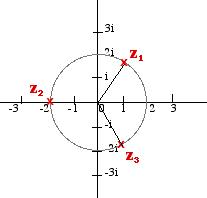We would be pleased if you find an error in the word problem, spelling mistakes, or inaccuracies and send it to us. Thank you!Tips to related online calculators
Try our complex numbers calculator.
Pythagorean theorem is the base for the right triangle calculator.

#### You need to know the following knowledge to solve this word math problem:

We encourage you to watch this tutorial video on this math problem:

## Next similar math problems:

• Complex number coordinatesWhich coordinates show the location of -2+3iA domed stadium is in the shape of spherical segment with a base radius of 150 m. The dome must contain a volume of 3500000 m³. Determine the height of the dome at its centre to the nearest tenth of a meter.
• Is complexAre these numbers 2i, 4i, 2i + 1, 8i, 2i + 3, 4 + 7i, 8i, 8i + 4, 5i, 6i, 3i complex?
• Imaginary numbersFind two imaginary numbers whose sum is a real number. How are the two imaginary numbers related? What is its sum?
• De Moivre's formula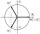There are two distinct complex numbers z such that z3 is equal to 1 and z is not equal 1. Calculate the sum of these two numbers.
• Log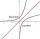Calculate value of expression log |3 +7i +5i2| .
• Im>0?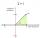Is -10i a positive number?
• ReciprocalCalculate reciprocal of z=0.8-1.8i:
• ABS CNCalculate the absolute value of complex number -15-29i.
• Linear imaginary equationGiven that ? "this is z star" Find the value of the complex number z.
• BearingA plane flew 50 km on a bearing 63°20' and the flew on a bearing 153°20' for 140km. Find the distance between the starting point and the ending point.
• Goniometric form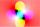Determine goniometric form of a complex number ?.
• The modulus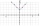Find the modulus of the complex number 2 + 5i
• Let z1=x1+y1i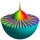Let z1=x1+y1i and z2=x2+y2i Find: a = Im (z1z2) b = Re (z1/z2)